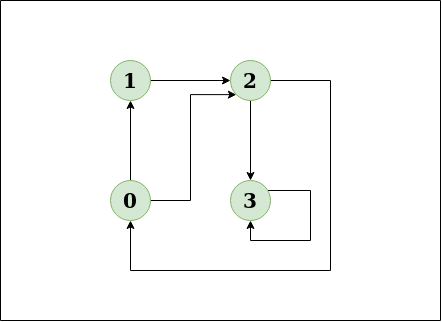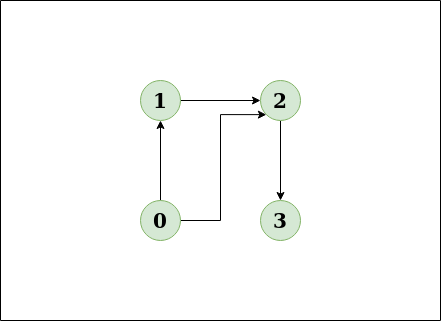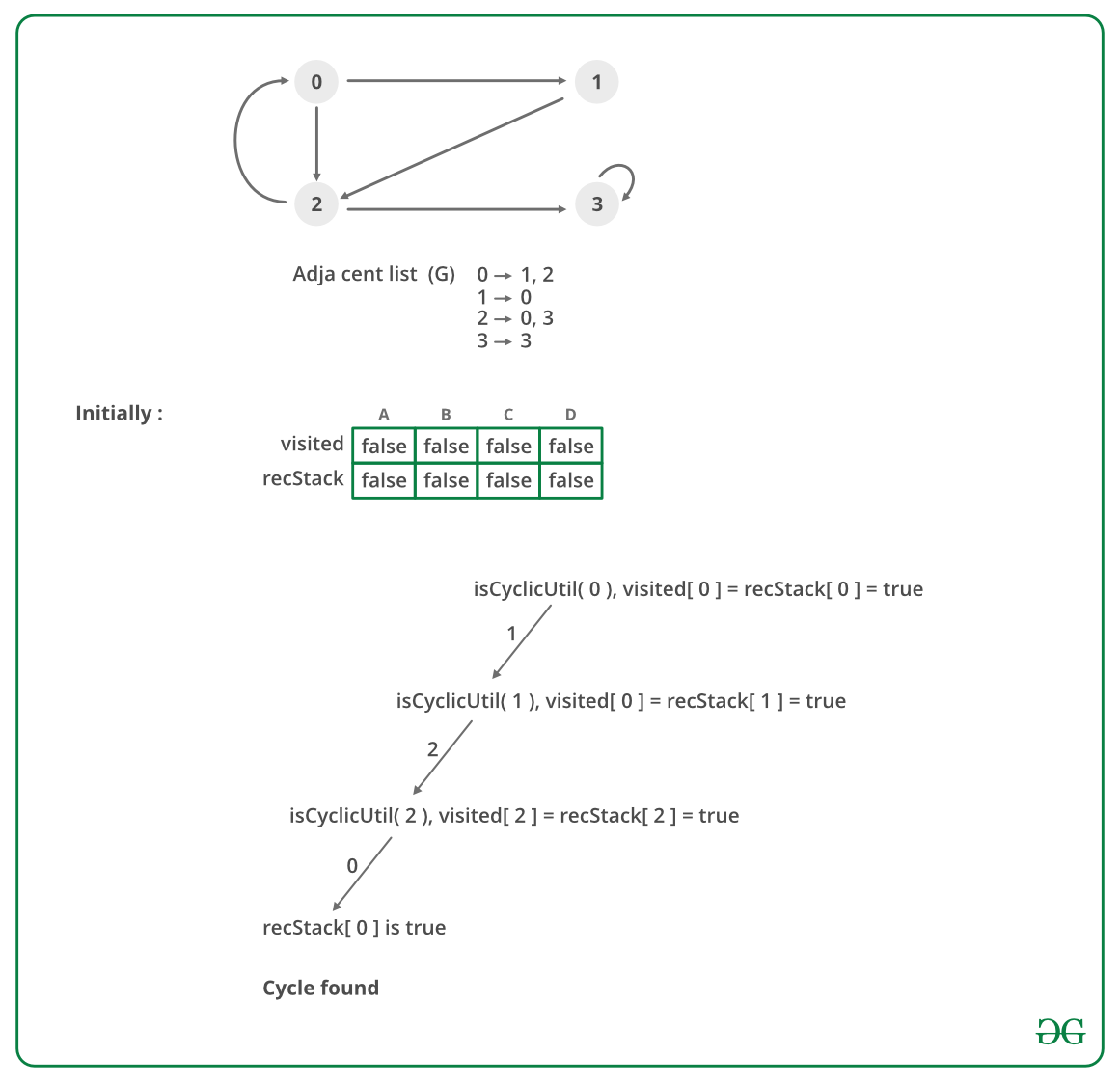Skip to content
Related Articles
Detect Cycle in a Directed Graph
• Difficulty Level : Medium
• Last Updated : 02 Jun, 2021

Given a directed graph, check whether the graph contains a cycle or not. Your function should return true if the given graph contains at least one cycle, else return false.
Example,

```Input: n = 4, e = 6
0 -> 1, 0 -> 2, 1 -> 2, 2 -> 0, 2 -> 3, 3 -> 3
Output: Yes
Explanation:
Diagram:``````The diagram clearly shows a cycle 0 -> 2 -> 0

Input:n = 4, e = 4
0 -> 1, 0 -> 2, 1 -> 2, 2 -> 3
Output:No
Explanation:
Diagram:````The diagram clearly shows no cycle`

Solution using Depth First Search or DFS

• Approach: Depth First Traversal can be used to detect a cycle in a Graph. DFS for a connected graph produces a tree. There is a cycle in a graph only if there is a back edge present in the graph. A back edge is an edge that is from a node to itself (self-loop) or one of its ancestors in the tree produced by DFS. In the following graph, there are 3 back edges, marked with a cross sign. We can observe that these 3 back edges indicate 3 cycles present in the graph.• For a disconnected graph, Get the DFS forest as output. To detect cycle, check for a cycle in individual trees by checking back edges.
To detect a back edge, keep track of vertices currently in the recursion stack of function for DFS traversal. If a vertex is reached that is already in the recursion stack, then there is a cycle in the tree. The edge that connects the current vertex to the vertex in the recursion stack is a back edge. Use recStack[] array to keep track of vertices in the recursion stack.
Dry run of the above approach:• Algorithm:
1. Create the graph using the given number of edges and vertices.
2. Create a recursive function that initializes the current index or vertex, visited, and recursion stack.
3. Mark the current node as visited and also mark the index in recursion stack.
4. Find all the vertices which are not visited and are adjacent to the current node. Recursively call the function for those vertices, If the recursive function returns true, return true.
5. If the adjacent vertices are already marked in the recursion stack then return true.
6. Create a wrapper class, that calls the recursive function for all the vertices and if any function returns true return true. Else if for all vertices the function returns false return false.
• Implementation:

•

## C++

 `// A C++ Program to detect cycle in a graph``#include` `using` `namespace` `std;` `class` `Graph``{``    ``int` `V;    ``// No. of vertices``    ``list<``int``> *adj;    ``// Pointer to an array containing adjacency lists``    ``bool` `isCyclicUtil(``int` `v, ``bool` `visited[], ``bool` `*rs);  ``// used by isCyclic()``public``:``    ``Graph(``int` `V);   ``// Constructor``    ``void` `addEdge(``int` `v, ``int` `w);   ``// to add an edge to graph``    ``bool` `isCyclic();    ``// returns true if there is a cycle in this graph``};` `Graph::Graph(``int` `V)``{``    ``this``->V = V;``    ``adj = ``new` `list<``int``>[V];``}` `void` `Graph::addEdge(``int` `v, ``int` `w)``{``    ``adj[v].push_back(w); ``// Add w to v’s list.``}` `// This function is a variation of DFSUtil() in https://www.geeksforgeeks.org/archives/18212``bool` `Graph::isCyclicUtil(``int` `v, ``bool` `visited[], ``bool` `*recStack)``{``    ``if``(visited[v] == ``false``)``    ``{``        ``// Mark the current node as visited and part of recursion stack``        ``visited[v] = ``true``;``        ``recStack[v] = ``true``;` `        ``// Recur for all the vertices adjacent to this vertex``        ``list<``int``>::iterator i;``        ``for``(i = adj[v].begin(); i != adj[v].end(); ++i)``        ``{``            ``if` `( !visited[*i] && isCyclicUtil(*i, visited, recStack) )``                ``return` `true``;``            ``else` `if` `(recStack[*i])``                ``return` `true``;``        ``}` `    ``}``    ``recStack[v] = ``false``;  ``// remove the vertex from recursion stack``    ``return` `false``;``}` `// Returns true if the graph contains a cycle, else false.``// This function is a variation of DFS() in https://www.geeksforgeeks.org/archives/18212``bool` `Graph::isCyclic()``{``    ``// Mark all the vertices as not visited and not part of recursion``    ``// stack``    ``bool` `*visited = ``new` `bool``[V];``    ``bool` `*recStack = ``new` `bool``[V];``    ``for``(``int` `i = 0; i < V; i++)``    ``{``        ``visited[i] = ``false``;``        ``recStack[i] = ``false``;``    ``}` `    ``// Call the recursive helper function to detect cycle in different``    ``// DFS trees``    ``for``(``int` `i = 0; i < V; i++)``        ``if` `(isCyclicUtil(i, visited, recStack))``            ``return` `true``;` `    ``return` `false``;``}` `int` `main()``{``    ``// Create a graph given in the above diagram``    ``Graph g(4);``    ``g.addEdge(0, 1);``    ``g.addEdge(0, 2);``    ``g.addEdge(1, 2);``    ``g.addEdge(2, 0);``    ``g.addEdge(2, 3);``    ``g.addEdge(3, 3);` `    ``if``(g.isCyclic())``        ``cout << ``"Graph contains cycle"``;``    ``else``        ``cout << ``"Graph doesn't contain cycle"``;``    ``return` `0;``}`

## Java

 `// A Java Program to detect cycle in a graph``import` `java.util.ArrayList;``import` `java.util.LinkedList;``import` `java.util.List;` `class` `Graph {``    ` `    ``private` `final` `int` `V;``    ``private` `final` `List> adj;` `    ``public` `Graph(``int` `V)``    ``{``        ``this``.V = V;``        ``adj = ``new` `ArrayList<>(V);``        ` `        ``for` `(``int` `i = ``0``; i < V; i++)``            ``adj.add(``new` `LinkedList<>());``    ``}``    ` `    ``// This function is a variation of DFSUtil() in``    ``// https://www.geeksforgeeks.org/archives/18212``    ``private` `boolean` `isCyclicUtil(``int` `i, ``boolean``[] visited,``                                      ``boolean``[] recStack)``    ``{``        ` `        ``// Mark the current node as visited and``        ``// part of recursion stack``        ``if` `(recStack[i])``            ``return` `true``;` `        ``if` `(visited[i])``            ``return` `false``;``            ` `        ``visited[i] = ``true``;` `        ``recStack[i] = ``true``;``        ``List children = adj.get(i);``        ` `        ``for` `(Integer c: children)``            ``if` `(isCyclicUtil(c, visited, recStack))``                ``return` `true``;``                ` `        ``recStack[i] = ``false``;` `        ``return` `false``;``    ``}` `    ``private` `void` `addEdge(``int` `source, ``int` `dest) {``        ``adj.get(source).add(dest);``    ``}` `    ``// Returns true if the graph contains a``    ``// cycle, else false.``    ``// This function is a variation of DFS() in``    ``// https://www.geeksforgeeks.org/archives/18212``    ``private` `boolean` `isCyclic()``    ``{``        ` `        ``// Mark all the vertices as not visited and``        ``// not part of recursion stack``        ``boolean``[] visited = ``new` `boolean``[V];``        ``boolean``[] recStack = ``new` `boolean``[V];``        ` `        ` `        ``// Call the recursive helper function to``        ``// detect cycle in different DFS trees``        ``for` `(``int` `i = ``0``; i < V; i++)``            ``if` `(isCyclicUtil(i, visited, recStack))``                ``return` `true``;` `        ``return` `false``;``    ``}` `    ``// Driver code``    ``public` `static` `void` `main(String[] args)``    ``{``        ``Graph graph = ``new` `Graph(``4``);``        ``graph.addEdge(``0``, ``1``);``        ``graph.addEdge(``0``, ``2``);``        ``graph.addEdge(``1``, ``2``);``        ``graph.addEdge(``2``, ``0``);``        ``graph.addEdge(``2``, ``3``);``        ``graph.addEdge(``3``, ``3``);``        ` `        ``if``(graph.isCyclic())``            ``System.out.println(``"Graph contains cycle"``);``        ``else``            ``System.out.println(``"Graph doesn't "``                                    ``+ ``"contain cycle"``);``    ``}``}` `// This code is contributed by Sagar Shah.`

## Python

 `# Python program to detect cycle``# in a graph` `from` `collections ``import` `defaultdict` `class` `Graph():``    ``def` `__init__(``self``,vertices):``        ``self``.graph ``=` `defaultdict(``list``)``        ``self``.V ``=` `vertices` `    ``def` `addEdge(``self``,u,v):``        ``self``.graph[u].append(v)` `    ``def` `isCyclicUtil(``self``, v, visited, recStack):` `        ``# Mark current node as visited and``        ``# adds to recursion stack``        ``visited[v] ``=` `True``        ``recStack[v] ``=` `True` `        ``# Recur for all neighbours``        ``# if any neighbour is visited and in``        ``# recStack then graph is cyclic``        ``for` `neighbour ``in` `self``.graph[v]:``            ``if` `visited[neighbour] ``=``=` `False``:``                ``if` `self``.isCyclicUtil(neighbour, visited, recStack) ``=``=` `True``:``                    ``return` `True``            ``elif` `recStack[neighbour] ``=``=` `True``:``                ``return` `True` `        ``# The node needs to be poped from``        ``# recursion stack before function ends``        ``recStack[v] ``=` `False``        ``return` `False` `    ``# Returns true if graph is cyclic else false``    ``def` `isCyclic(``self``):``        ``visited ``=` `[``False``] ``*` `(``self``.V ``+` `1``)``        ``recStack ``=` `[``False``] ``*` `(``self``.V ``+` `1``)``        ``for` `node ``in` `range``(``self``.V):``            ``if` `visited[node] ``=``=` `False``:``                ``if` `self``.isCyclicUtil(node,visited,recStack) ``=``=` `True``:``                    ``return` `True``        ``return` `False` `g ``=` `Graph(``4``)``g.addEdge(``0``, ``1``)``g.addEdge(``0``, ``2``)``g.addEdge(``1``, ``2``)``g.addEdge(``2``, ``0``)``g.addEdge(``2``, ``3``)``g.addEdge(``3``, ``3``)``if` `g.isCyclic() ``=``=` `1``:``    ``print` `"Graph has a cycle"``else``:``    ``print` `"Graph has no cycle"` `# Thanks to Divyanshu Mehta for contributing this code`

## C#

 `// A C# Program to detect cycle in a graph``using` `System;``using` `System.Collections.Generic;` `public` `class` `Graph {``    ` `    ``private` `readonly` `int` `V;``    ``private` `readonly` `List> adj;` `    ``public` `Graph(``int` `V)``    ``{``        ``this``.V = V;``        ``adj = ``new` `List>(V);``        ` `        ``for` `(``int` `i = 0; i < V; i++)``            ``adj.Add(``new` `List<``int``>());``    ``}``    ` `    ``// This function is a variation of DFSUtil() in``    ``// https://www.geeksforgeeks.org/archives/18212``    ``private` `bool` `isCyclicUtil(``int` `i, ``bool``[] visited,``                                    ``bool``[] recStack)``    ``{``        ` `        ``// Mark the current node as visited and``        ``// part of recursion stack``        ``if` `(recStack[i])``            ``return` `true``;` `        ``if` `(visited[i])``            ``return` `false``;``            ` `        ``visited[i] = ``true``;` `        ``recStack[i] = ``true``;``        ``List<``int``> children = adj[i];``        ` `        ``foreach` `(``int` `c ``in` `children)``            ``if` `(isCyclicUtil(c, visited, recStack))``                ``return` `true``;``                ` `        ``recStack[i] = ``false``;` `        ``return` `false``;``    ``}` `    ``private` `void` `addEdge(``int` `sou, ``int` `dest) {``        ``adj[sou].Add(dest);``    ``}` `    ``// Returns true if the graph contains a``    ``// cycle, else false.``    ``// This function is a variation of DFS() in``    ``// https://www.geeksforgeeks.org/archives/18212``    ``private` `bool` `isCyclic()``    ``{``        ` `        ``// Mark all the vertices as not visited and``        ``// not part of recursion stack``        ``bool``[] visited = ``new` `bool``[V];``        ``bool``[] recStack = ``new` `bool``[V];``        ` `        ` `        ``// Call the recursive helper function to``        ``// detect cycle in different DFS trees``        ``for` `(``int` `i = 0; i < V; i++)``            ``if` `(isCyclicUtil(i, visited, recStack))``                ``return` `true``;` `        ``return` `false``;``    ``}` `    ``// Driver code``    ``public` `static` `void` `Main(String[] args)``    ``{``        ``Graph graph = ``new` `Graph(4);``        ``graph.addEdge(0, 1);``        ``graph.addEdge(0, 2);``        ``graph.addEdge(1, 2);``        ``graph.addEdge(2, 0);``        ``graph.addEdge(2, 3);``        ``graph.addEdge(3, 3);``        ` `        ``if``(graph.isCyclic())``            ``Console.WriteLine(``"Graph contains cycle"``);``        ``else``            ``Console.WriteLine(``"Graph doesn't "``                                    ``+ ``"contain cycle"``);``    ``}``}` `// This code contributed by Rajput-Ji`
• Output:

`Graph contains cycle`
•
• Complexity Analysis:
• Time Complexity: O(V+E).
Time Complexity of this method is same as time complexity of DFS traversal which is O(V+E).
• Space Complexity: O(V).
To store the visited and recursion stack O(V) space is needed.

In the below article, another O(V + E) method is discussed :
Detect Cycle in a direct graph using colors
Please write comments if you find anything incorrect, or you want to share more information about the topic discussed above.

Attention reader! Don’t stop learning now. Get hold of all the important DSA concepts with the DSA Self Paced Course at a student-friendly price and become industry ready.  To complete your preparation from learning a language to DS Algo and many more,  please refer Complete Interview Preparation Course.

In case you wish to attend live classes with industry experts, please refer Geeks Classes Live

My Personal Notes arrow_drop_up